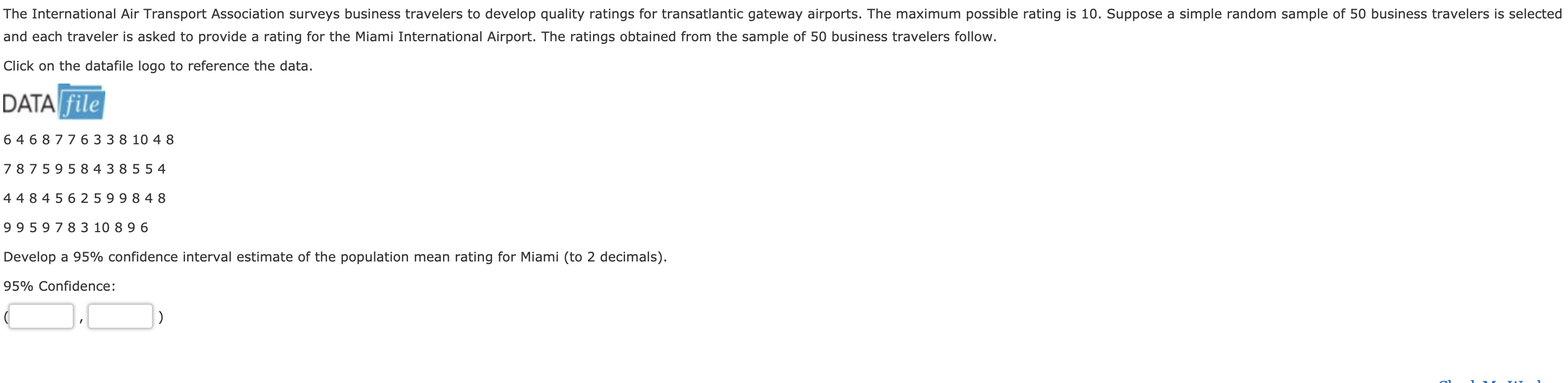The International Air Transport Association surveys business travelers to develop quality ratings for transatlantic gateway airports. The maximum possible rating is 10. Suppose a simple random sample of 50 business travelers is selectedand each traveler is asked to provide a rating for the Miami International Airport. The ratings obtained from the sample of 50 business travelers follow.Click on the datafile logo to reference the data.DATA file6 468 776 33 8 10 4 87 8759584 3 855 44484 5625 99 8 489959 7 8 3 10 89 6Develop a 95% confidence interval estimate of the population mean rating for Miami (to 2 decimals).95% Confidence

Questionhelp_outlineImage TranscriptioncloseThe International Air Transport Association surveys business travelers to develop quality ratings for transatlantic gateway airports. The maximum possible rating is 10. Suppose a simple random sample of 50 business travelers is selected and each traveler is asked to provide a rating for the Miami International Airport. The ratings obtained from the sample of 50 business travelers follow. Click on the datafile logo to reference the data. DATA file 6 468 776 33 8 10 4 8 7 8759584 3 855 4 4484 5625 99 8 48 9959 7 8 3 10 89 6 Develop a 95% confidence interval estimate of the population mean rating for Miami (to 2 decimals). 95% Confidence fullscreen
Step 1

Obtain the mean and standard deviation of ratings for the random sample of 50 business travelers of Miami:

Excel procedure to find the mean and standard deviation:

Step 1: Enter the values of ratings from A2:A51.

Step 2: Obtain the mean in cell A52, by entering the formula “=AVERAGE(A2:A51)”.

Step5: Obtain the standard deviation in cell A53, by entering the formula “=STDEVA(A2:A51)”.

Step 2

Confidence interval estimate of population mean using t–distribution:

The required conditions for using t distribution to estimate the confidence interval about mean is given below:

• The population standard deviation σ must be unknown.
• Either the sample size (n) must be greater than 30 or the population must be normally distributed.

Here, the population standard deviation is unknown and the sample size of ratings is 50 (>30).

The confidence interval estimate of population mean using t–distribution is,

Step 3

Obtain the critical value:

The required confidence level is 100*(1–α)% = 95%.

From the right tailed t-values of t-...

Want to see the full answer?

See Solution

Want to see this answer and more?

Our solutions are written by experts, many with advanced degrees, and available 24/7

See Solution
Tagged in

Hypothesis Testing